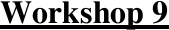# BUSS1020 Study Guide - Midterm Guide: Test Statistic, Descriptive Statistics, Statistical Hypothesis Testing

42 views4 pagesWorkshop 9
Hypothesis Testing
Learning Objectives:
At the end of this workshop, students should be able to:
1. Understand the type of hypotheses that we test and how to select the null and alternative
hypotheses.
2. Understand the difference between a one-tail test and a two-tail test.
3. Understand the types of errors we can make when we test hypotheses.
4. Use the 6 step procedure to undertake a hypothesis test for a mean, when the population variance
is known.
5. Use the 6 step procedure to undertake a hypothesis test for a mean, when the population variance
is unknown.
6. Calculate the p-value for a test statistic relating to means and proportions.
7. Use the 5 step p-value procedure to undertake a hypothesis test for a mean, whether the
population variance is known or unknown.
8. Write a one or two sentence report (other than reject H0 ) relating the conclusion back to the
1. Read the question and write down all the given information. Note if you are given the sample or
population mean and the sample or population standard deviation.
2. State your hypotheses including the level of significance.
3. State the distribution you are using.
4. Calculate the rejection region.
5. Calculate the value of the test statistic.
6. Write your conclusions in words.
Calculating the rejection region.
1. Draw your diagram of the normal distribution.
2. Ask the question “Is it a 1 or 2 tail test? Is it a right hand tail or a left hand tail or both?
3. How do you know if it is a 1 or 2 tail test? Look at the sign in the alternative hypothesis. If the sign
is > or < then it is a 1 tail test. If the sign is z, then it is a 2 tail test.
5. Now use your normal tables to work out where the rejection region starts.
6. Note: The rejection region is the area in the tails (either 1 or 2 tails).
Unlock document

This preview shows page 1 of the document.
Unlock all 4 pages and 3 million more documents.